• pytorch 张量乘积和矩阵乘法
千次阅读
2021-02-07 17:27:17

pytorch 张量的乘积和矩阵乘法

## 张量的乘积( tensor 维度需要一致，对应 位置 元素相乘 )

tensor = torch.ones(4,4)
tensor[:,1] = 2
print('tensor:')
print(tensor)

# 逐个元素相乘结果
print(f"tensor.mul(tensor): \n {tensor.mul(tensor)} \n")
# 等价写法:
print(f"tensor * tensor: \n {tensor * tensor}")



输出：

tensor:
tensor([[1., 2., 1., 1.],
[1., 2., 1., 1.],
[1., 2., 1., 1.],
[1., 2., 1., 1.]])
tensor.mul(tensor):
tensor([[1., 4., 1., 1.],
[1., 4., 1., 1.],
[1., 4., 1., 1.],
[1., 4., 1., 1.]])

tensor * tensor:
tensor([[1., 4., 1., 1.],
[1., 4., 1., 1.],
[1., 4., 1., 1.],
[1., 4., 1., 1.]])


## 矩阵乘法（tensor 维度需要一致）

tensor = torch.ones(4,4)
tensor[:,1] = 2
print('tensor:')
print(tensor)

print(f"tensor.matmul(tensor.T): \n {tensor.matmul(tensor.T)} \n")
# 等价写法:
print(f"tensor @ tensor.T: \n {tensor @ tensor.T}")


输出：

tensor:
tensor([[1., 2., 1., 1.],
[1., 2., 1., 1.],
[1., 2., 1., 1.],
[1., 2., 1., 1.]])
tensor.matmul(tensor.T):
tensor([[7., 7., 7., 7.],
[7., 7., 7., 7.],
[7., 7., 7., 7.],
[7., 7., 7., 7.]])

tensor @ tensor.T:
tensor([[7., 7., 7., 7.],
[7., 7., 7., 7.],
[7., 7., 7., 7.],
[7., 7., 7., 7.]])


更多相关内容
• 根据 De Lathauwer 的定义并被许多论文引用。... I_N ) 的张量U: R^( J × I_n) 的矩阵B：R^(I_1 × I_2 × .. J × .. I_N) 的输出张量n：[1:N]内的标量，指定模式 句法： B = nmodeproduct(A, U, n)matlab
• ## 张量(矩阵)乘积

万次阅读 2018-05-14 15:25:58
1 矩阵乘积 (matmul product) 设A为m*p的矩阵，B为p*n 的矩阵，那么称m*n的矩阵C为矩阵A与B的乘积， 记作 C = AB 其中矩阵C中的第 行第 列元素可以表示为： 这里写代码片 2 哈达马积 (hadamard product) m*...

关于张量里面的积有太多形式了,这里终结一下,并用Python实现
1 矩阵乘积 (matmul product)
Am*p的矩阵，Bp*n 的矩阵，那么称m*n的矩阵C为矩阵A与B的乘积，
记作 C = AB
其中矩阵C中的第 行第 列元素可以表示为：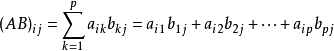这里写代码片

m*n矩阵 A 与m*n矩阵 B 的Hadamard积记为A*B.
其元素定义为两个矩阵对应元素的乘积: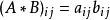这里写代码片

3 克罗内克积 (Kronecker product)
Kronecker积是两个任意大小的矩阵间的运算，表示为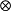,又称为直积或张量积.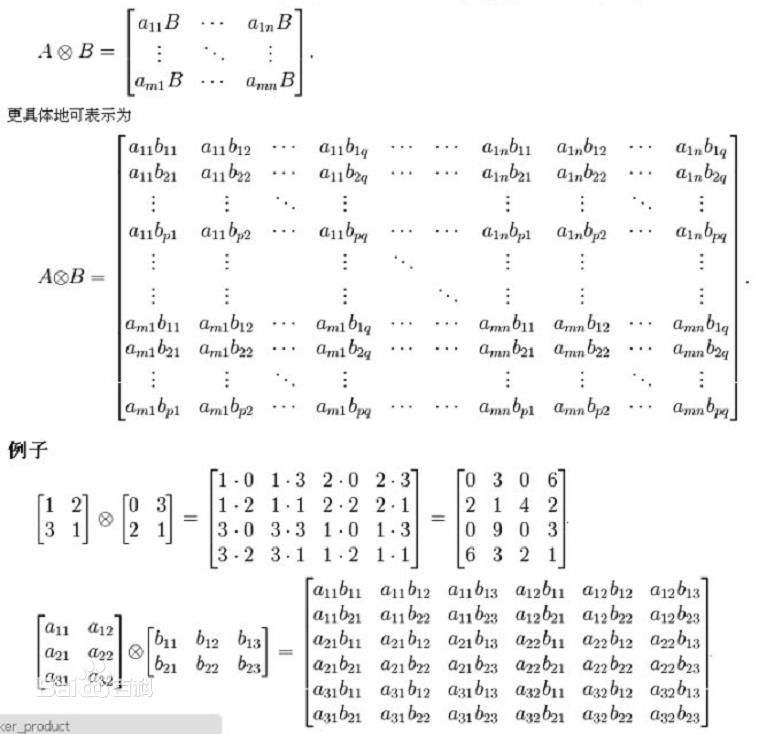展开全文Python tensor
• 总和是在 a b 的索引上完成的，这些索引不是 c 的索引 “点”在不使用额外内存的情况下就地工作：不使用“repmat”或“托尼的技巧”。 由于使用了“ddot”（BLAS） MEX 文件，“dots”相当快。 该功能与 ...matlab
• matlab张量积代码矩阵乘积状态Langevin （1）在MATLAB中针对有限自旋链实现矩阵乘积状态Langevin方程，以及（2）对于无限自旋链实现时间相关的变分原理（）。 矩阵乘积状态Langevin方程描述了与环境热接触的系统的...
• @TOC 一、Tensor-train分解 1. 定义 给定N阶张量，将其分解成N个二阶或三阶张量的缩并形式: 2. TT低秩近似 二、矩阵乘积态 1. 定义 2. 矩阵乘积态与量子纠缠 3. 矩阵乘积态的规范自由度与正交形式

### TT分解和矩阵乘积态

我是一只正在不断学习、希望早日成为小白的小小白，有什么错误欢迎大家批评指正，喜欢的请点个赞哦！

# 一、Tensor-train分解（TT分解）

## 1. 定义

给定N阶张量，将其分解成N个二阶或三阶张量的缩并形式:

T S 1 S 2 … S N = ∑ a 1 a 2 … a N − 1 A S 1 a 1 ( 1 ) A S 2 a 1 a 2 ( 2 ) … A S N − 1 a N − 2 a N − 1 ( N − 1 ) A S N ( N ) = A S 1 : ( 1 ) A S 2 : ⋯ ( 2 ) … A S N − 1 : : ( N − 1 ) A S N : ( N ) T T_{S_{1} S_{2} \ldots S_{N}}=\sum_{a_{1} a_{2} \ldots a_{N-1}} A_{S_{1} a_{1}}^{(1)} A_{S_{2} a_{1} a_{2}}^{(2)} \ldots A_{S_{N-1} a_{N-2} a_{N-1}}^{(N-1)} A_{S_{N}}^{(N)}=A_{S_{1}:}^{(1)} A_{S_{2}: \cdots}^{(2)} \ldots A_{S_{N-1}::}^{(N-1)} A_{S_{N}:}^{(N) \mathrm{T}}

其中， { a n } \left\{ {{a}_{n}} \right\} 被称为几何或辅助指标， { s n } \left\{ {{s}_{n}} \right\} 被称为开放或物理指标。

tensor-train直译过来就是张量火车，正如下图我们所看到的那样，一个大的张量被分解成了一个个小张量缩并的形式，就像我们平时所看到的一节节火车的车厢，所以不得不说前人还是很富有想象力的。为了方便，在后面的学习中我们会把Tensor-train分解简称为TT分解。TT分解可以通过N-1次奇异值分解实现。先把第一个指标看成一个指标，后面三个指标看成一个大指标，这样子就得到一个二阶张量（矩阵），将其进行奇异值分解，左边就是奇异值分解后得到的 U U ，右边就是 Λ V † \Lambda {{V}^{\dagger }} 。同理对右边的张量按照上述方法继续进行奇异值分解……例如四阶张量的TT分解，如下图：经过N-1次奇异值分解，一个N阶的张量就被分解成了N个小张量。但是严格的TT分解不能解决指数墙的问题，辅助指标会随着奇异值分解的次数呈指数增长。那么这个时候怎么解决这个问题呢？可以先思考下，答案后面揭晓。## 2. TT低秩近似

TT秩的定义
每一次进行奇异值分解所得到的非0奇异值的个数（辅助指标的维数），因为N阶张量要进行N-1次奇异值分解，所以一个N阶张量的TT秩包含N-1个数。TT低秩近似
令辅助指标有一个上限，辅助指标的上限 χ \chi 称为截断维数或裁剪维数:
min ⁡ { dim ⁡ ( a n ) ≤ χ } ∣ T s 1 s 2 … s N − ∑ a 1 a 1 … a N − 1 A s 1 a 1 ( 1 ) A s 2 ( 2 ) a 1 a 2 ⋯ A s N − 1 a N − 2 a N − 1 ( N − 1 ) A S N a N − 1 ( N ) \min _{\left\{\operatorname{dim}\left(a_{n}\right) \leq \chi\right\}} \mid T_{s_{1} s_{2} \ldots s_{N}}-\sum_{a_{1} a_{1} \ldots a_{N-1}} A_{s_{1} a_{1}}^{(1)} A_{s_{2}}^{(2)} a_{1} a_{2} \cdots A_{s_{N-1} a_{N-2} a_{N-1}}^{(N-1)} A_{S_{N} a_{N-1}}^{(N)}
获得TT秩近似最简单的算法之一就是每次进行奇异值分解后将多于截断维数的奇异值和对应的奇异向量进行裁剪，将辅助指标的维数裁剪为 χ \chi ，简而言之就是在进行TT分解时不断利用奇异值分解的低秩近似，是不是超级容易就解决了一个问题。

随机生成10×10×10×10的张量进行不同截断维数的TT低秩近似的误差（下图为 χ \chi 取30到60）附上代码，没事可以自己去试试，感觉有点神奇呢！（看我这没见过世面的样子U•ェ•U）：

import numpy as np
import BasicFun as bf
import matplotlib.pyplot as plt

# 考虑(2, 2, 2, 2, 2, 2, 2, 2, 2, 2)的8阶张量，进行无裁剪TT分解，查看分解后辅助指标的维数
x = np.random.randn(2, 2, 2, 2, 2, 2, 2, 2, 2, 2)
tensors = bf.ttd(x)
# 读取辅助指标维数
a_dims = list()
for x in tensors[:-1]:
a_dims.append(x.shape[-1])
print('The ancillary dimensions of the TT form = ')
print(a_dims)

# 考虑(10, 10, 10, 10)的张量，计算不同截断维数下的误差
y = np.random.randn(10, 10, 10,10)
err = list()
z0 = np.linalg.norm(y)
for chi in range(30, 61,1):
tensors = bf.ttd(y, chi)
y1 = bf.tt_product(tensors)
err.append(np.linalg.norm(y - y1) / z0)
print('\nThe error with different dimension cut-off = ')
print(err)
plt.plot(np.arange(30, 61,1), err, marker='x')
plt.xlabel(r'dimension cut-off $\chi$')
plt.ylabel('relative error')
plt.show()


# 二、矩阵乘积态（MPS）

## 1. 定义

一般而言，矩阵乘积态（MPS）定义为系数矩阵满足TT形式的量子态:
∣ φ ⟩ = ∑ s 1 s 2 … s N φ s 1 s 2 … s N ∏ ⊗ n = 1 N ∣ s n ⟩ |\varphi\rangle=\sum_{s_{1} s_{2} \ldots s_{N}} \varphi_{s_{1} s_{2} \ldots s_{N}} \prod_{\otimes n=1}^{N}\left|s_{n}\right\rangle
在不引起误解的情况下矩阵乘积态也可以直接理解为系数矩阵:
φ s 1 s 2 … s N = ∑ a 1 a 1 … a N − 1 A s 1 a 1 ( 1 ) A s 2 a 1 a 2 ( 2 ) … A s N − 1 a N − 2 a N − 1 ( N − 1 ) A S N a N − 1 ( N ) = A s 1 : ( 1 ) A S 2 : ⋯ ( 2 ) … A S N − 1 : ( N − 1 ) A S N : ( N ) T \varphi_{s_{1} s_{2} \ldots s_{N}}=\sum_{a_{1} a_{1} \ldots a_{N-1}} A_{s_{1} a_{1}}^{(1)} A_{s_{2} a_{1} a_{2}}^{(2)} \ldots A_{s_{N-1} a_{N-2} a_{N-1}}^{(N-1)} A_{S_{N} a_{N-1}}^{(N)}=A_{s_{1}:}^{(1)} A_{S_{2}: \cdots}^{(2)} \ldots A_{S_{N-1}:}^{(N-1)} A_{S_{N}:}^{(N) \mathrm{T}}

矩阵乘积态的两种边界条件分别为开发边界条件（OBC）和周期边界条件（PBC），如下图：在TT分解中，量子态参数个数随N指数增加，满足:
⁣ ⁣ #  ⁣ ⁣   ( ∣ φ ⟩ ) ∼ O ( d N ) \text{ }\!\!\#\!\!\text{ }\left( \left| \varphi \right\rangle \right)\sim O\left( {{d}^{N}} \right)
在MPS中，给定辅助指标截断维数为 ，则MPS包含参数的个数随N线性增加，满足：
⁣ ⁣ #  ⁣ ⁣   ( M P S ) ∼ O ( N d χ 2 ) \text{ }\!\!\#\!\!\text{ }\left( MPS \right)\sim O\left( Nd{{\chi }^{2}} \right)
对于MPS分解，我们并不需要知道指数复杂的量子系数是什么，也不需要进行TT分解，而是直接假设基态具备MPS的形式，直接处理MPS中的局部张量，从而绕开指数墙的问题，但是这样子会引入误差。并且，在求基态时我们是在并不知道基态是什么的情况下直接假设基态是具有给定截断维数的MPS态，因此我们并不能确定这样的MPS态可以有效的描述基态。我们可以从奇异谱入手定义刻画MPS的有效性，而MPS态对应的奇异谱刚好就是量子态的量子纠缠谱，由此引入量子纠缠。有没有很懵，don’t worry，继续往下看！## 2. 矩阵乘积态与量子纠缠

斯密特分解与纠缠谱：给定N自旋的量子态
∣ φ ⟩ = ∑ s 1 s 2 ⋯ s N φ s 1 s 2 ⋯ s N ∏ ⊗ n = 1 N ∣ s n ⟩ \left| \varphi \right\rangle =\sum\nolimits_{{{s}_{1}}{{s}_{2}}\cdots {{s}_{N}}}{{{\varphi }_{{{s}_{1}}{{s}_{2}}\cdots {{s}_{N}}}}\prod\limits_{\otimes n=1}^{N}{\left| {{s}_{n}} \right\rangle }}

将自旋分成两部分:
{ s n } = ( s 1 , s 2 , ⋯   , s K ) ∪ ( s K + 1 , ⋯   , s N ) \left\{ {{s}_{n}} \right\}=\left( {{s}_{1}},{{s}_{2}},\cdots ,{{s}_{K}} \right)\cup ({{s}_{K+1}},\cdots ,{{s}_{N}})

两部分不能为空，将前面一部分看成一个指标，后面一部分看成一个指标，这样就得到一个二阶张量（矩阵），对矩阵的系数进行奇异值分解：
φ s 1 s 2 ⋯ s N = ∑ a = 0 D − 1 U s 1 s 2 ⋯ s K , a Λ a V s K + 1 ⋯ s N , a ∗ {{\varphi }_{{{s}_{1}}{{s}_{2}}\cdots {{s}_{N}}}}=\sum\limits_{a=0}^{D-1}{{{U}_{{{s}_{1}}{{s}_{2}}\cdots {{s}_{K}},a}}{{\Lambda }_{a}}}V_{{{s}_{K+1}}\cdots {{s}_{N}},a}^{*}

对系数进行奇异值分解对应于对量子态做分解：
∣ φ ⟩ = ∑ a = 0 D − 1 Λ a ∣ U a ⟩ ∣ V a ⟩ \left| \varphi \right\rangle =\sum\limits_{a=0}^{D-1}{{{\Lambda }_{a}}\left| {{U}^{a}} \right\rangle \left| {{V}^{a}} \right\rangle }

∣ U a ⟩ ∣ V a ⟩ \left| {{U}^{a}} \right\rangle \left| {{V}^{a}} \right\rangle 之间进行直积， Λ a {{\Lambda }_{a}} 可看成权重系数。该分解被称为量子态的斯密特分解，量子态的斯密特分解对应于系数矩阵的奇异值分解， Λ \Lambda 称为量子态的纠缠谱。其中 ∣ U a ⟩ ∣ V a ⟩ \left| {{U}^{a}} \right\rangle \left| {{V}^{a}} \right\rangle 为D个量子态，满足：
∣ U a ⟩ = ∑ s 1 s 2 ⋯ s K D − 1 U s 1 s 2 ⋯ s K , a ∏ ⊗ n = 1 K ∣ s n ⟩ \left| {{U}^{a}} \right\rangle =\sum\limits_{{{s}_{1}}{{s}_{2}}\cdots {{s}_{K}}}^{D-1}{{{U}_{{{s}_{1}}{{s}_{2}}\cdots {{s}_{K}},a}}\prod\limits_{\otimes n=1}^{K}{\left| {{s}_{n}} \right\rangle }}

∣ V a ⟩ = ∑ s 1 s 2 ⋯ s K D − 1 V ∗ s K + 1 ⋯ s N , a ∏ ⊗ n = 1 K ∣ s n ⟩ \left| {{V}^{a}} \right\rangle =\sum\limits_{{{s}_{1}}{{s}_{2}}\cdots {{s}_{K}}}^{D-1}{{{V}^{*}}_{{{s}_{K+1}}\cdots {{s}_{N}},a}\prod\limits_{\otimes n=1}^{K}{\left| {{s}_{n}} \right\rangle }}

由于
⟨ φ ∣ φ ⟩ =1 ， ∣ φ ⟩ = ∑ a = 0 D − 1 Λ a ∣ U a ⟩ ∣ V a ⟩ \left\langle \varphi \right|\left. \varphi \right\rangle \text{=1}，\left| \varphi \right\rangle \text{=}\sum\limits_{a=0}^{D-1}{{{\Lambda }_{a}}\left| {{U}^{a}} \right\rangle \left| {{V}^{a}} \right\rangle }

所以
⟨ φ ∣ φ ⟩ = ∑ a = 0 D − 1 Λ a 2 ⟨ V a ∣ ⟨ U a ∣ U a ⟩ ∣ V a ⟩ = ∑ a = 0 D − 1 Λ a 2 =1 \left\langle \varphi \right|\left. \varphi \right\rangle \text{=}\sum\limits_{a=0}^{D-1}{{{\Lambda }_{a}}^{\text{2}}\left\langle {{V}^{a}} \right|\left\langle {{U}^{a}} \right.\left| {{U}^{a}} \right\rangle \left| {{V}^{a}} \right\rangle }\text{=}\sum\limits_{a=0}^{D-1}{{{\Lambda }_{a}}^{\text{2}}\text{=1}}

定义 ∣ φ ⟩ \left| \varphi \right\rangle 被投影到 ∣ U a ⟩ ∣ V a ⟩ \left| {{U}^{a}} \right\rangle \left| {{V}^{a}} \right\rangle 态的概率满足 P a = Λ a 2 {{P}_{a}}=\Lambda _{a}^{2} （量子力学的基本假设），显然概率满足归一化条件
∑ a P a = 1 \sum\nolimits_{a}{{{P}_{a}}=1}

根据概率论香农熵的定义：

E S = − P a ∑ a ln ⁡ P a {{E}^{S}}=-{{P}_{a}}\sum\nolimits_{a}{\ln {{P}_{a}}}

根据该公式定义量子态的纠缠熵：

S = − ∑ a = 0 D − 1 Λ a 2 ln ⁡ Λ a 2 S=-\sum\limits_{a=0}^{D-1}{\Lambda _{a}^{2}}\ln \Lambda _{a}^{2}

因为香农熵是刻画信息量的大小，类推到量子态，那么量子态的纠缠熵就是刻画量子信息量的大小。把量子态二分为A体系和B体系，A、B体系在进行给定基底下的概率测量得到的信息熵就是这两个体系在该二分下的纠缠熵。

奇异值分解中
φ s 1 s 2 ⋯ s N = ∑ a = 0 D − 1 U s 1 s 2 ⋯ s K , a Λ a V s K + 1 ⋯ s N , a ∗ {{\varphi }_{{{s}_{1}}{{s}_{2}}\cdots {{s}_{N}}}}=\sum\limits_{a=0}^{D-1}{{{U}_{{{s}_{1}}{{s}_{2}}\cdots {{s}_{K}},a}}{{\Lambda }_{a}}}V_{{{s}_{K+1}}\cdots {{s}_{N}},a}^{*}

U V UV 满足正交性：
U † U = ∑ s 1 … S K U s 1 … S K , α ∗ U S 1 … S K , α ′ = I U^{\dagger} U=\sum_{s_{1}\ldots S_{K}} U_{s_{1} \ldots S_{K}, \alpha}^{*} U_{S_{1} \ldots S_{K}, \alpha^{\prime}}=I

V † V = ∑ s 1 … S K V s 1 … S K , α ∗ V S 1 … S K α ′ = I V^{\dagger} V=\sum_{s_{1}\ldots S_{K}} V_{s_{1} \ldots S_{K}, \alpha}^{*} V_{S_{1} \ldots S_{K} \alpha^{\prime}}=I

由上述性质可计算开放边界MPS的纠缠，设MPS态满足：
φ s 1 s 2 … s N = ∑ a 1 a 1 … a N − 1 A s 1 a 1 ( 1 ) … A s K a K − 1 a K ( K ) Λ a K ( K ) A s K + 1 a K a K + 1 ( K + 1 ) … A S N a N − 1 ( N ) \varphi_{s_{1} s_{2} \ldots s_{N}}=\sum_{a_{1} a_{1} \ldots a_{N-1}} A_{s_{1} a_{1}}^{(1)} \ldots A_{s_{K} a_{K-1} a_{K}}^{(K)} \Lambda_{a_{K}}^{(K)} A_{s_{K+1} a_{K} a_{K+1}}^{(K+1)} \ldots A_{S_{N} a_{N-1}}^{(N)}

当如下左/右正交条件满足时， Λ K {{\Lambda }^{K}} 为MPS给出的前K个自旋与其余自旋之间的纠缠：

a. ∑ s 1 A s 1 a 1 ( 1 ) A s 1 a 1 ( 1 ) ∗ = I a 1 a 1 \sum_{s_{1}} A_{s_{1} a_{1}}^{(1)} A_{s_{1} a_{1}}^{(1) *}=I_{a_{1} a_{1}}
b. ∑ s n a n − 1 A s n a n − 1 a n ( n ) A s n a n − 1 a n ( n ) ∗ = I a n a n ( 1 < n < K ) \sum_{s_{n} a_{n-1}} A_{s_{n} a_{n-1} a_{n}}^{(n)} A_{s_{n} a_{n-1} a_{n}}^{(n) *}=I_{a_{n} a_{n}} \quad(1<n<K)
c . ∑ s N A s N a N − 1 ( N ) A S N a N − 1 ( N ) ∗ = I a N − 1 a N − 1 \mathrm{c.} \sum_{s_{N}} A_{s_{N} a_{N-1}}^{(N)} A_{S_{N} a_{N-1}}^{(N) *}=I_{a_{N-1} a_{N-1}}
d. ⁡ ∑ s n a n + 1 A s n a n − 1 a n ( n ) A s n a n − 1 a n ( n ) ∗ = I a n − 1 a n − 1 ( K < n < N ) \operatorname{d.} \sum_{s_{n} a_{n+1}} A_{s_{n} a_{n-1} a_{n}}^{(n)} A_{s_{n} a_{n-1} a_{n}}^{(n) *}=I_{a_{n-1} a_{n-1}}(K<n<N)
e. Λ 0 ( K ) ≥ Λ 1 ( K ) ≥ ⋯ ≥ 0 \Lambda_{0}^{(K)} \geq \Lambda_{1}^{(K)} \geq \cdots \geq 0

前面K个tensor收缩构成SVD分解中的U，后面的tensor收缩构成SVD分解中的V，称为MPS的中心正交形式，也称为SVD形式。例如长度为4的MPS，箭头表示正交条件的方向：MPS的中心正交形式在后面的学习中会经常涉及，且与SVD分解紧密联系在一起，所以这个要好好进行理解消化(　o=^•ェ•)o！

## 3. 矩阵乘积态的规范自由度与正交形式

计算MPS的斯密特分解可将MPS变成SVD形式，但是其所表示的量子态不能改变。改变MPS中的tensor但不改变其所表示的量子态，被称为规范变换也就是物理量不改变、数学形式改变的变化。进一步定义MPS的规范自由度：对于同一个量子态，可由多组由不同张量组成的MPS态来表示其系数。

已知MPS满足：
φ S 1 S 2 … S N = A S 1 : ( 1 ) … A S n : ( n ) A S n + 1 : ⋯ ( n + 1 ) … A S N : ( N ) T \varphi_{S_{1} S_{2} \ldots S_{N}}=A_{S_{1}:}^{(1)} \ldots A_{S_{n}:}^{(n)} A_{S_{n+1}: \cdots}^{(n+1)} \ldots A_{S_{N}:}^{(N) \mathrm{T}}

引入任意可逆矩阵 U U 及其逆矩阵 U − 1 {{U}^{-1}} ，定义:
B s n ( n ) = A s n : : ( n ) U , B s n ( n + 1 ) = U − 1 A s n : : ( n + 1 ) B_{{{s}_{n}}}^{(n)}=A_{{{s}_{n}}::}^{(n)}U,B_{{{s}_{n}}}^{(n+1)}={{U}^{-1}}A_{{{s}_{n}}::}^{(n+1)}

得到同一个量子态的两种MPS表示:
φ s 1 s 2 … s N = A s 1 ( 1 ) … A s n : : ( n ) A s n + 1 : ⋯ ( n + 1 ) … A s N : ( N ) T = A s 1 : ( 1 ) … B s n : ( n ) B s n + 1 ! ( n + 1 ) … A S N : ( N ) T \varphi_{s_{1} s_{2} \ldots s_{N}}=A_{s_{1}}^{(1)} \ldots A_{s_{n}::}^{(n)} A_{s_{n+1}: \cdots}^{(n+1)} \ldots A_{s_{N}:}^{(N) \mathrm{T}}=A_{s_{1}:}^{(1)} \ldots B_{s_{n}:}^{(n)} B_{s_{n+1} !}^{(n+1)} \ldots A_{S_{N}:}^{(N) \mathrm{T}}

公式总是没有图来得直观，下面我们用下图来进行进一步理解。MPS规范变换可以在任何一个或多个辅助指标上插入一个单位阵，也就是任何可逆矩阵及其逆矩阵的乘积。我们都知道任何矩阵乘单位阵都等于自己本身，所以在任何一个或多个辅助指标上插入一个单位阵都不会改变量子态。将变换矩阵作用到各张量{ A ( n ) {{A}^{(n)}} }上，就能得到新张量{ B ( n ) {{B}^{(n)}} }在这种情况下，我们插入不同维数的单位矩阵就会得到不同的新张量{ B ( n ) {{B}^{(n)}} }，这个时候，我们可以通过引入新的约束条件，固定MPS的规范自由度，使得给定量子态具备唯一的MPS表示。

例如MPS的中心正交形式，如下图所示。当张量{ A ( n ) {{A}^{(n)}} }（n<k）满足左正交条件，{ A ( n ) {{A}^{(n)}} }（n>k）满足右正交条件，中间的tensor满足归一条件，此时MPS被称为具有 K K -中心正交形式。可通过多次的SVD分解进行规范变换从 K K -中心正交形式变换成K‘-中心正交形式（ K ≠ K ′ K\ne K' ），这个过程值得细细思考品味。给定任意一个矩阵乘积态，求量子纠缠谱（ Λ ( K ) {{\Lambda }^{(K)}} ）：
（1） 把该矩阵乘积态变成K-中心正交形式；
（2） 对K-中心正交形式的中心tensor做奇异值分解。

基于K-中心正交形式，可对MPS辅助指标维数进行最优裁剪，裁剪方法为：
（1） 进行中心正交化，将正交中心放置于第K个张量；
（2） 对中心张量进行奇异值分解
A s K a K − 1 a K ( K ) = ∑ β = 0 χ − 1 U s K a K − 1 β Λ β ( K ) V a K β A_{{{s}_{K}}{{a}_{K-1}}{{a}_{K}}}^{(K)}=\sum\nolimits_{\beta =0}^{\chi -1}{{{U}_{{{s}_{K}}{{a}_{K-1}}\beta }}\Lambda _{\beta }^{(K)}{{V}_{{{a}_{K}}\beta }}}

仅保留前 χ \chi 个奇异值及对应的奇异值向量，其中 χ \chi 为截断维数；
（3） 将第K个张量更新为 U → A ( K ) U\to {{A}^{(K)}} ;
（4） 将第（K+1）个张量更新为
∑ a K Λ β ( K ) V a K β A s K + 1 a K a K + 1 ( K + 1 ) → A s K + 1 β a K + 1 ( K + 1 ) \sum\nolimits_{{{a}_{K}}}{\Lambda _{\beta }^{(K)}{{V}_{{{a}_{K}}\beta }}}A_{{{s}_{K+1}}{{a}_{K}}{{a}_{K+1}}}^{(K+1)}\to A_{{{s}_{K+1}}\beta {{a}_{K+1}}}^{(K+1)}

通过不断移动正交中心的位置来实现MPS辅助指标的最优裁剪。

MPS的正则形式：给定量子态
∣ φ ⟩ = ∑ s 1 s 2 ⋯ s N φ s 1 s 2 ⋯ s N ∏ ⊗ n = 1 N ∣ s n ⟩ \left| \varphi \right\rangle =\sum\nolimits_{{{s}_{1}}{{s}_{2}}\cdots {{s}_{N}}}{{{\varphi }_{{{s}_{1}}{{s}_{2}}\cdots {{s}_{N}}}}\prod\limits_{\otimes n=1}^{N}{\left| {{s}_{n}} \right\rangle }}

其系数满足：
φ S 1 S 2 … S N = A S 1 : ( 1 ) Λ ( 1 ) A S 2 : Λ ( 2 ) Λ ( 2 ) … Λ ( N − 2 ) A S N − 1 : Λ ( N − 1 ) Λ ( N − 1 ) A S N : ( N ) T \varphi_{S_{1} S_{2} \ldots S_{N}}=A_{S_{1}:}^{(1)} \Lambda^{(1)} A_{S_{2}: \Lambda}^{(2)} \Lambda^{(2)} \ldots \Lambda^{(N-2)} A_{S_{N-1}: \Lambda}^{(N-1)} \Lambda^{(N-1)} A_{S_{N}:}^{(N) \mathrm{T}}

Λ ( n ) {{\Lambda }^{(n)}} 是当前位置下进行二分得到的纠缠谱，满足:

a. ∑ s 1 A s 1 a 1 ( 1 ) A s 1 a 1 ( 1 ) ∗ = I a 1 a 1 \sum_{s_{1}} A_{s_{1} a_{1}}^{(1)} A_{s_{1} a_{1}}^{(1) *}=I_{a_{1} a_{1}}
b. ∑ s n a n − 1 Λ a n − 1 a n − 1 ( n − 1 ) A s n a n − 1 a n ( n ) Λ a n − 1 a n − 1 ( n − 1 ) A s n a n − 1 a n ( n ) ∗ = I a n a n \sum_{s_{n} a_{n-1}} \Lambda_{a_{n-1} a_{n-1}}^{(n-1)} A_{s_{n} a_{n-1} a_{n}}^{(n)} \Lambda_{a_{n-1} a_{n-1}}^{(n-1)} A_{s_{n} a_{n-1} a_{n}}^{(n) *}=I_{a_{n} a_{n}}
c. ∑ s n a n A s n a n − 1 a n ( n ) Λ a n a n ( n ) A s n a n − 1 a n ( n ) ∗ Λ a n a n ( n ) = I a n − 1 a n − 1 ( 1 < n < K ) \sum_{s_{n} a_{n}} A_{s_{n} a_{n-1} a_{n}}^{(n)} \Lambda_{a_{n} a_{n}}^{(n)} A_{s_{n} a_{n-1} a_{n}}^{(n) *} \Lambda_{a_{n} a_{n}}^{(n)}=I_{a_{n-1} a_{n-1}} \quad(1<n<K)
d. ∑ S N A S N ( N ) a N − 1 A S N a ′ N − 1 ( N ) ∗ = I a N − 1 a P − 1 \sum_{S_{N}} A_{S_{N}}^{(N)} a_{N-1} A_{S_{N} a^{\prime} N-1}^{(N) *}=I_{a_{N-1} a_{P-1}}有没有觉很很神奇，神奇的事情还在后面，我们下期见！
我是一只正在不断学习、希望早日成为小白的小小白，有什么错误欢迎大家批评指正，喜欢的请点个赞哦！展开全文python 量子力学 机器学习
• 我们提供各种时间演化方法，重点是通过矩阵乘积状态形式主义来支持远程交互。 有关更多算法，请参见下面的功能列表。 如果您的出版物涉及以下内容，请引用“ ML WallLD Carr，New J. Phys。14，125015（2012）”...开源软件
• 在Matlab中计算一个张量和一个矩阵的mode-n（模n）乘积，相关运算原理请查找相关教材。
• 对于手性CFT，近似值采用矩阵乘积状态的形式。 对于由手性反手性部分组成的完整CFT，近似值由有限相关状态给出。 我们表明，键维数在逼近误差的反函数中呈多项式缩放，而在最小dis的反函数中呈亚指数式增长
• 根据上一篇博客提到的N阶张量的TT分解，我们可以把这样的系数矩阵进行分解，，满足TT形式的量子态我们将其称为矩阵乘积态（MPS）。 写成TT形式后，我们可以通过前述截断辅助指标维数的方法来降低某一量子态的参数...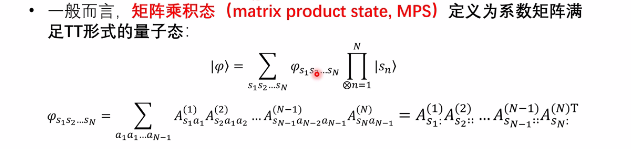首先，任意的复合量子态可以写成上面的基于基底的表述形式，他的系数 ψ \psi 实际上构成了一个N阶张量，si代表着第i个子系统的某个基矢，也代表着N阶张量的某一维度。根据上一篇博客提到的N阶张量的TT分解，我们可以把这样的系数矩阵进行分解，，满足TT形式的量子态我们将其称为矩阵乘积态（MPS）。写成TT形式后，我们可以通过前述截断辅助指标维数的方法来降低某一量子态的参数复杂度。TT形式中间的每一个张量，有一个物理指标（维数为ｄ）和两个辅助指标（维数被截断），总共我们有N个这样的张量，所以参数的复杂度是可求的。显然可见，我们通过这样的方法把参数复杂度从指数级降到了线性级。

但是，这里还存在一个问题，TT分解本质上是对已有的一个张量进行分解，如果我已经知道了这样的一个N阶参数矩阵，所谓的降低复杂度也没有了什么意义。所以，为了绕过指数墙，我们直接假设MPS态，直接求解局部张量。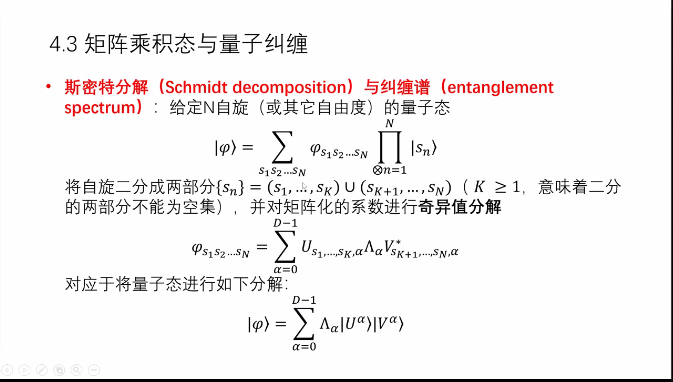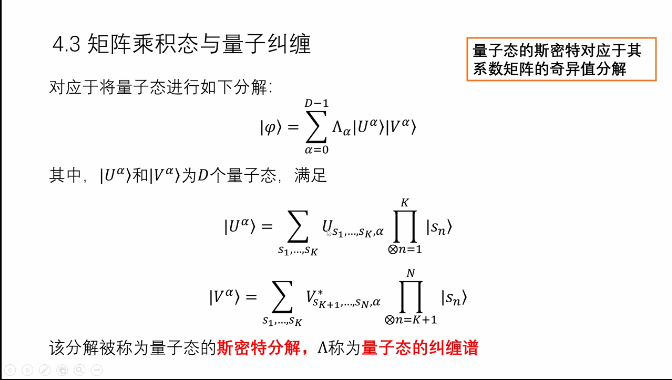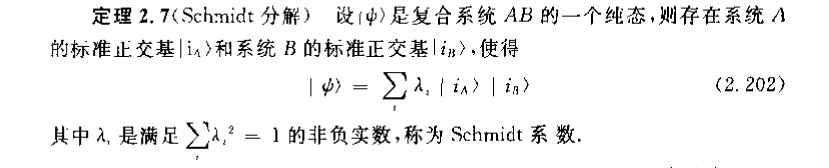由于是奇异值分解，所以，U和V都是量子态（归一），由 ∣ ψ > |\psi> 是归一的，可以得到 Λ \Lambda 平方和为1（U本身是一组正交归一的量子态，是前K个子系统复合后的系统的一组标准正交基）(其实，U和V的维数都是受到了奇异谱的限制，与相应的子系统的维数没啥关系），所以，设计的这一组U和V满足schmidt分解的要求。

这里的奇异谱在schmidt分解里面又称为纠缠谱，纠缠谱数目的多少称为schmidt数。某种意义上来说，schmidt数反映了两个系统之间的纠缠程度。当且仅当schmidt数等于1时， ψ \psi 可写成积状态也就是可分态，两子系统之间不存在纠缠性。另外，由纠缠谱可引伸出纠缠熵的概念。

另一个问题，我们直接设定局部张量，我们怎么知道这样得到的MPS态和真实的量子态之间的误差是多大？如果我的张量可以写成奇异值分解的形式，我就可以衡量两者之间的误差。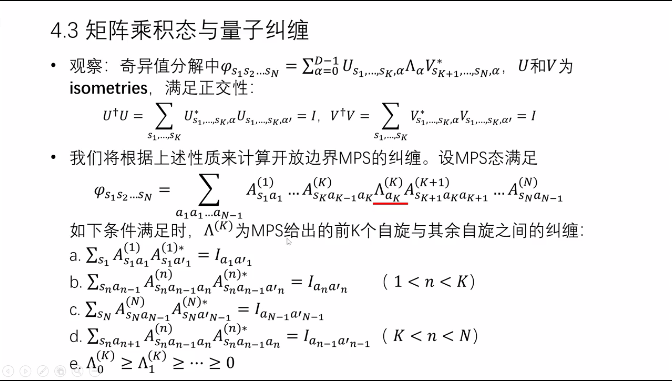这边是为了凑出奇异值分解的形式，如果要满足奇异值分解，则两边的矩阵应该是酉矩阵。而下方的a,b,c,d条件实际上在TT分解的过程中就应当保持的（在这里可理解成，左边分出了左特征向量矩阵，右边是把右特征向量矩阵分出来了）对比之前的TT分解图，这里的分解是有一定的区别的（在新增加的对角阵的右边）
在a,b,c,d条件下，大家可以自行证明 U × U ∗ U\times U^* = I I （证明的时候式子比较长，缩并的维度也比较多，要仔细一点），所以如果我们能把量子态写成这样的形式，同时满足a,b,c,d,e条件，那么这样的形式我们就可以称为MPS态的SVD形式。联系前面所提的schmidt分解的内容，这样SVD形式中的 Λ \Lambda 反映了前k个子系统与其余系统的纠缠关系。

展开全文• 要将tensor1的后两维构成的k个矩阵与tensor2中的矩阵矩阵乘法得到 shape 为[k, m, p]的向量   解决办法： 1，我们知道TensorFlow的matmul已经支持了batch，即： A = tf.Variable(tf.random_normal(...tensorflow
• 张量乘积的三种形式 ...1、矩阵乘积 (matmul product) 2 、哈达马积 (hadamard product) 3 、克罗内克积 (Kronecker product) ---------------------------------------------------------------------------java 学习 开发语言
• 例子向量化转置矩阵可用于将参数的顺序颠倒为其他非可交换 Kronecker 乘积。 >> m=2;n=3;p=4;q=2; >> A = rand(m,n); B = rand(p,q); >> isequal(TvecMat(p,m)*kron(A,B)*TvecMat(n,q),kron(B,A)) 答案 = 1 有关...matlab
• 我们可以理解为纬度[n,1]或者[1,n]，一维的矩阵。 在python中，我们设置一个array，查看其shape： >>> import numpy as np >>> a = np.array([1,2,3,4,5]) >>> a array([1, 2, 3, 4, 5])...python numpy
• 通过在矩阵变量后加’的方法来表示转置运算a=[10,2,12;34,2,4;98,34,6];a'%% ans =%% 10 34 98%% 2 2 34%% 12 4 6% 2. 矩阵求逆inv(a)% ans =%% -0.0116 0.0372 -0.0015%% 0.0176 -0.1047 0.0345%% 0.0901 -0.0135 ...
• 前两天我主要学习了卷积神经网络的一些相关理论，并写成了一篇博客，这一期 ，基于前面学习过张量矩阵乘积态的相关基础理论深度学习中的卷积神经网络，我们下面将学习论文中 是如何将这二者完美的结合在一起！...神经网络 算法 图像识别
• 编写不易如果觉得不错，麻烦关注一下~ 【1】参考连接https://blog.csdn.net/hopyGreat/article/details/86351972 【矩阵乘积】 【2】https://positive.blog.csdn.net/article/details/113744587?ivk_sa=1024320u...
• ## 向量、矩阵、张量基础知识

万次阅读 多人点赞 2018-07-31 19:05:43
学习并且做这篇笔记目的：学习张量，初步了解张量分解（tensor decomposition）领域。那么什么是张量分解呢？研究它具体的应用是什么？ 为了便于理解，我们先从矩阵分解讲起。 我们知道现在的数据大部分都是以矩阵...
• I have a 2D matrix M of shape [batch x dim], I have a vector V of shape [batch]. How can I multiply each of the columns in the matrix by the corresponding element in the V? That is:I know an inefficie...
• 1.矩阵乘积态 2.矩阵乘积态与量子纠缠 3.纠缠熵 4.中心正交形式（SVD形式）
• 文章目录一、向量张量矩阵1、向量2、矩阵3、多维向量二、向量与矩阵的运算1、范数2、向量的乘积3、矩阵相乘三、张量的运算四、矩阵的逆伪逆五、行列式六、线性方程组七、二次型与正定性八、矩阵分解 一、向量张量...机器学习 深度学习
• 用于张量运算的张量实用程序，如收缩、子张量提取、外积、张量排列和矩阵... 其中一些是将 Kronecker 乘积矩阵转置和矩阵乘法作为特殊情况的运算，而这里被推广到任意价的张量。 阅读函数标题以获取示例用户指南。matlab
• ITensor库还包括可组合和可扩展的算法，用于优化转换张量网络，例如矩阵乘积状态和矩阵乘积运算符，例如DMRG算法。 西蒙斯基金会下属的熨斗研究所（Flatiron Institute）支持ITensor的开发。 安装 ITensors...
• 循环矩阵与向量乘积的离散傅立叶变换 证明过程非常有意思写下来给大家看一下 证明： FAB=(FA(:,1))⊙(FB) FAB=(FA(:,1))\odot(FB) FAB=(FA(:,1))⊙(FB) 其中AAA为循环矩阵，F为DFT矩阵，A(:,1)A(:,1)A(:,1)是AAA的第...matlab 傅里叶变换...